# NCERT Solutions for Class 10 Maths Exercise 7.2## myCBSEguide App

Complete Guide for CBSE Students

NCERT Solutions, NCERT Exemplars, Revison Notes, Free Videos, CBSE Papers, MCQ Tests & more.

NCERT solutions for Maths Coordinate Geometry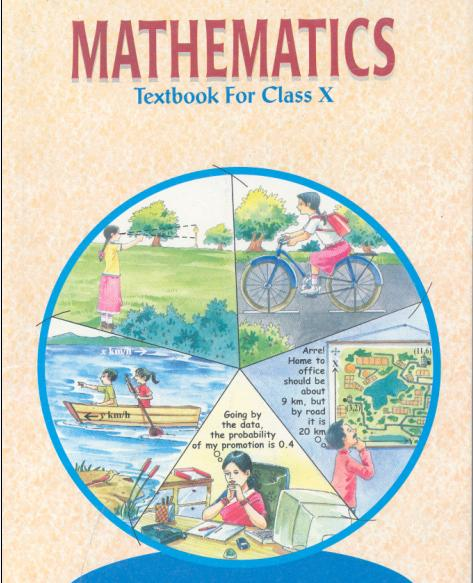## NCERT Solutions for Class 10 Maths Coordinate Geometry

###### 1. Find the coordinates of the point which divides the join of (–1, 7) and (4, –3) in the ratio 2:3.

Ans. Let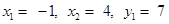and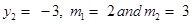Using Section Formula to find coordinates of point which divides join of (–1, 7) and (4, –3) in the ratio 2:3, we get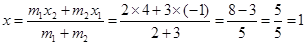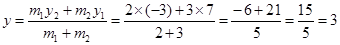Therefore, the coordinates of point are (1, 3) which divides join of (–1, 7) and

(4, –3) in the ratio 2:3.

NCERT Solutions for Class 10 Maths Exercise 7.2

###### 2. Find the coordinates of the points of trisection of the line segment joining (4, –1) and (–2, –3).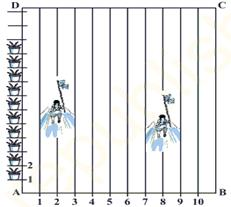Ans.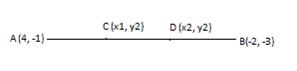We want to find coordinates of the points of trisection of the line segment joining (4, –1) and (–2, –3).

We are given AC = CD = DB

We want to find coordinates of point C and D.

Let coordinates of point C be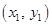and let coordinates of point D be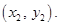Clearly, point C divides line segment AB in 1:2 and point D divides line segment AB in 2:1.

Using Section Formula to find coordinates of point C which divides join of (4, –1) and (–2, –3) in the ratio 1:2, we get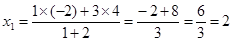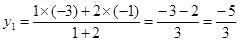Using Section Formula to find coordinates of point D which divides join of (4, –1) and (–2, –3) in the ratio 2:1, we get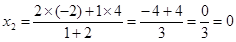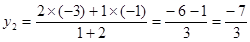Therefore, coordinates of point C are (2,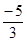) and coordinates of point D are (0,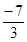).

NCERT Solutions for Class 10 Maths Exercise 7.2

###### 3. To conduct sports day activities, in your rectangular shaped school ground ABCD, lines have been drawn with chalk powder at a distance of 1 m each. 100 flower pots have been placed at a distance of 1 m from each other along AD. Niharika runs 14th of the distance AD on the 2nd line and posts a green flag. Preet runs 15th of the distance AD on the eighth line and posts a red flag. What is the distance between both the flags? If Rashmi has to post a blue flag exactly halfway between the line segment joining the two flags, where should she post her flag?

Ans. Niharika runs 14th of the distance AD on the 2nd line and posts a green flag.

There are 100 flower pots. It means, she stops at 25th flower pot.

Therefore, the coordinates of point where she stops are (2 m, 25 m).

Preet runs 15th of the distance AD on the eighth line and posts a red flag. There are 100 flower pots. It means, she stops at 20th flower pot.

Therefore, the coordinates of point where she stops are (8, 20).

Using Distance Formula to find distance between points (2 m, 25 m) and (8 m, 20 m), we get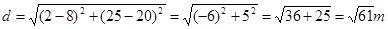Rashmi posts a blue flag exactly halfway the line segment joining the two flags.

Using section formula to find the coordinates of this point, we get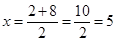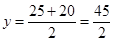Therefore, coordinates of point, where Rashmi posts her flag are (5,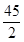).

It means she posts her flag in 5th line after covering= 22.5 m of distance.

NCERT Solutions for Class 10 Maths Exercise 7.2

###### 4. Find the ratio in which the line segment joining the points (–3, 10) and (6, –8) is divided by (–1, 6).

Ans. Let (–1, 6) divides line segment joining the points (–3, 10) and (6, –8) in k:1.

Using Section formula, we get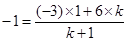⇒ −k – 1 = (−3 + 6k)

⇒ −7k = −2

⇒ k =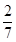Therefore, the ratio is:1 which is equivalent to 2:7.

Therefore, (–1, 6) divides line segment joining the points (–3, 10) and (6, –8) in 2:7.

NCERT Solutions for Class 10 Maths Exercise 7.2

###### 5. Find the ratio in which the line segment joining A (1, –5) and B (–4, 5) is divided by the x–axis. Also find the coordinates of the point of division.

Ans. Let the coordinates of point of division be (x, 0) and suppose it divides line segment joining A (1, –5) and B (–4, 5) in k:1.

According to Section formula, we get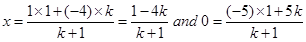… (1)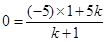⇒ 5 = 5k

⇒ k = 1

Putting value of k in (1), we get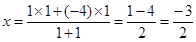Therefore, point (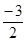, 0) on x–axis divides line segment joining A (1, –5) and B (–4, 5) in 1:1.

NCERT Solutions for Class 10 Maths Exercise 7.2

###### 6. If (1, 2), (4, y), (x, 6) and (3, 5) are the vertices of a parallelogram taken in order, find x and y.

Ans. Let A = (1, 2), B = (4, y), C = (x, 6) and D = (3, 5)

We know that diagonals of parallelogram bisect each other. It means that coordinates of midpoint of diagonal AC would be same as coordinates of midpoint of diagonal BD. … (1)

Using Section formula, the coordinates of midpoint of AC are: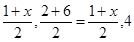Using Section formula, the coordinates of midpoint of BD are: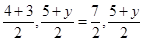According to condition (1), we have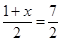⇒ (1 + x) = 7

⇒ x = 6

Again, according to condition (1), we also have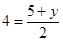⇒ 8 = 5 + y

⇒ y = 3

Therefore, x = 6 and y = 3

NCERT Solutions for Class 10 Maths Exercise 7.2

###### 7. Find the coordinates of a point A, where AB is the diameter of a circle whose centre is (2, –3) and B is (1, 4).

Ans. We want to find coordinates of point A. AB is the diameter and coordinates of center are (2, –3) and, coordinates of point B are (1, 4).

Let coordinates of point A are (x, y). Using section formula, we get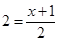⇒ 4 = x + 1

⇒ x = 3

Using section formula, we get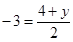⇒ −6 = 4 + y

⇒ y = −10

Therefore, Coordinates of point A are (3, –10).

NCERT Solutions for Class 10 Maths Exercise 7.2

###### 8. If A and B are (–2, –2) and (2, –4) respectively, find the coordinates of P such that AP =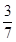AB and P lies on the line segment AB.

Ans. A = (–2, –2) and B = (2, –4)It is given that AP=AB

PB = AB – AP = AB −AB =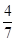AB

So, we have AP: PB = 3: 4

Let coordinates of P be (x, y)

Using Section formula to find coordinates of P, we get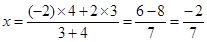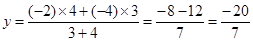Therefore, Coordinates of point P are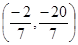.

NCERT Solutions for Class 10 Maths Exercise 7.2

###### 9. Find the coordinates of the points which divides the line segment joining A (–2, 2) and B (2, 8) into four equal parts.

Ans. A = (–2, 2) and B = (2, 8)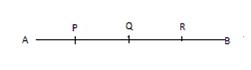Let P, Q and R are the points which divide line segment AB into 4 equal parts.

Let coordinates of point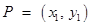,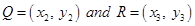We know AP = PQ = QR = RS.

It means, point P divides line segment AB in 1:3.

Using Section formula to find coordinates of point P, we get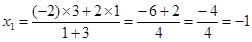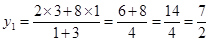Since, AP = PQ = QR = RS.

It means, point Q is the mid-point of AB.

Using Section formula to find coordinates of point Q, we get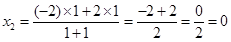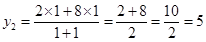Because, AP = PQ = QR = RS.

It means, point R divides line segment AB in 3:1

Using Section formula to find coordinates of point P, we get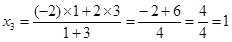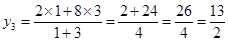Therefore, P = (–1,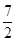), Q= (0,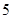)and R = (1,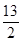)

NCERT Solutions for Class 10 Maths Exercise 7.2

###### 10. Find the area of a rhombus if its vertices are (3, 0), (4, 5), (–1, 4) and (–2, –1) taken in order.{Hint: Area of a rhombus =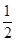(product of its diagonals)}

Ans. Let A = (3, 0), B = (4, 5), C = (–1, 4) and D = (–2, –1)

Using Distance Formula to find length of diagonal AC, we get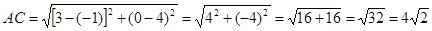Using Distance Formula to find length of diagonal BD, we get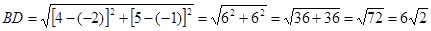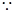Area of rhombus = ½ (product of its diagonals)

=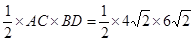= 24 sq. units

## NCERT Solutions for Class 10 Maths Exercise 7.2

NCERT Solutions Class 10 Maths PDF (Download) Free from myCBSEguide app and myCBSEguide website. Ncert solution class 10 Maths includes text book solutions from Mathematics Book. NCERT Solutions for CBSE Class 10 Maths have total 15 chapters. 10 Maths NCERT Solutions in PDF for free Download on our website. Ncert Maths class 10 solutions PDF and Maths ncert class 10 PDF solutions with latest modifications and as per the latest CBSE syllabus are only available in myCBSEguide.

## CBSE app for Class 10

To download NCERT Solutions for Class 10 Maths, Computer Science, Home Science,Hindi ,English, Social Science do check myCBSEguide app or website. myCBSEguide provides sample papers with solution, test papers for chapter-wise practice, NCERT solutions, NCERT Exemplar solutions, quick revision notes for ready reference, CBSE guess papers and CBSE important question papers. Sample Paper all are made available through the best app for CBSE## Test Generator

Create Papers with your Name & Logo

### 8 thoughts on “NCERT Solutions for Class 10 Maths Exercise 7.2”

1. Very nice
I like this website

2. poda dai onnum puriyala

3. Thanks

4. Nice very easy to understand

5. sahi hai

6. Thanks it really help me

7. It’s nice

8. Good app